##1. Triangle conics

By this term I mean conics circumscribing a triangle ABC (i.e. passing through its vertices) or inscribed in a triangle (i.e. tangent to its side-lines). Such conics go always in pairs: one inscribed (c1 in the figure) paired with one circumscribed (c0 in the figure) and the relation between the two conics is very natural and based on the idea of trilinear polar tr(P) (see TrilinearPolar.html ) of a point P with respect to ABC. Point P and the trilinear polar LP=tr(P) of P are called respectively perspector and perspectrix of the conic (both c0 and c1). For definitions and first elementary properties of the ideas used see the file Trilinears.html as well as IsogonalGeneralized.html .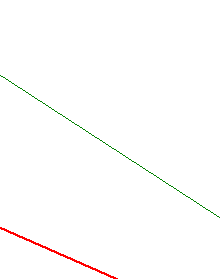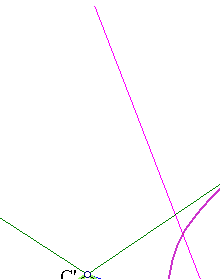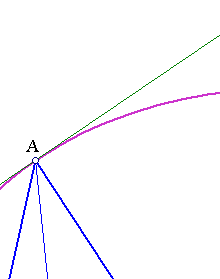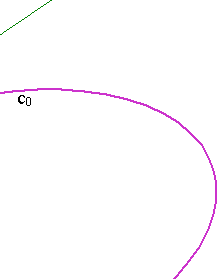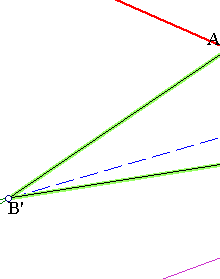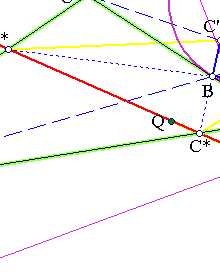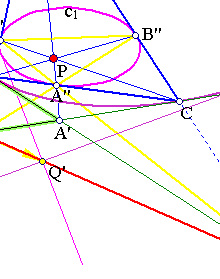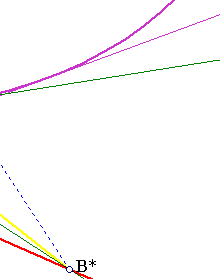To study the properties of the two conics a very convenient coordinate system is the one defined by the projective base {A,B,C,P}, in which these points have respectively the coordinates (1,0,0), (0,1,0), (0,0,1) and (1,1,1) (see ProjectiveBase.html ).

 The traces of P, points {A'',B'',C''} have respectively the representations {A''=B+C, B''=C+A, C''=A+B}.
 The harmonic associates of P, points {A',B',C'} have respectively the representations {A'=B+C-A, B'=C+A-B, C'=A+B}.
 The conjugates of A'',B'',C'' with respect to the vertices of the triangle, which are on the tripolar tr(P) of P have correspondingly the representation {A*=B-C, B*=C-A, C*=A-B-C}.
 The trilinear polar LP=tr(P) of P has the equation x+y+z = 0.
 More general, the trilinear polar of any point Q(a,b,c)=aA+bB+cC has the equation (1/a)x+(1/b)y+(1/c)z = 0.
 Conic c0 has the equation yz+zx+xy = 0.
 The equation of the conic c1 is easily calculated considering it as a member of the bitangent family generated by lines AB (represented by z=0), AC (represented by y=0) and C''B'' (represented by x-y-z=0).
The conic has then the form yz - k(x-y-z)2 = 0, and using that A'' is on this conic, k is seen to be 1/4.
Finally conic c1 obtains the form -x2-y2-z2+2xy+2yz+2zx=0.
 The matrices C1 of c0 and C2 of c0 are adjoint to each other (each a multiple of the other's inverse):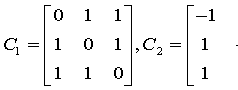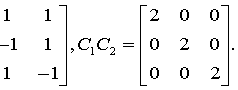This shows that each is the dual of the other i.e. it can be identified with the conic representing the tangents of the other (in the respective projective space).
 The family generated by the two conics: {k1c0 + k2c1} contains the member 4c0 - c1 = (x+y+z)2 = 0, which is the trilinear polar Lp of P (considered as a degenerate double-line-conic).##2. General properties

 Conic c0 is the locus of tripoles of lines through P.
In fact, such a line ax+by+cz=0 has a+b+c=0 (since P(1,1,1) is on it), hence its tripole S(1/a,1/b,1/c) satisfies equation [1.6].
 The tangents of c1 are tripolars tr(Q) of points Q on the tripolar tr(P).
The calculation is contained in section 8 of IsogonalGeneralized.html .
 The tangents of c0 are tripolars tr'(Q) (tr' denoting tripolars w.r. to A'B'C') of points Q on the tripolar tr(P).
Apply  since c0 has to A'B'C' the relation c1 has to ABC.
 For every point Q on tr(P) the two tripolars tr(Q) and tr'(Q) intersect on tr(P). Line tr(P) is the polar of P with respect to both conics c0 and c1.
This is discussed in IsotomicGeneral.html .
Introduce the generalized isogonal transformation which in the coordinates of section 1 has the form:
F(x,y,z) = (1/x,1/y,1/z).
 Circumconic c0 is the image F(tr(P)) of the tripolar of P.
 Inconic c1 is the image F''(tr(P)) under the corresponding isogonal transformation for triangle A''B''C''.
From the two last assertions the first is obvious and the second reduces to the first.
 The two conics c0, c1 have no points in common, their matrices are inverse (up to a factor) to each other and generate a family of conics in which tr(P) is contained as a double line.
This follows from the calculations of [1.8].
A quick proof of these properties can be also given by noticing that the two conics are images of the circum/in-circle of an equilateral under an appropriate homography F. Homography F is defined by the requirements (i) to map the vertices of the equilateral to the vertices of the triangle, (ii) to map the center of the equilateral to point P. The line at infinity is then mapped under F onto LP=tr(P). This method is discussed in IsotomicGeneral.html .

InconicsTangents.html
IncircleTangents2.html
InconicsTangents.html
IsogonalGeneralized.html
IsotomicGeneral.html
ProjectiveBase.html
PascalOnTriangles.html
ProjectiveCoordinates.html
ProjectivityFixingVertices.html
Steiner_Ellipse.html
TriangleCircumconics.html
TriangleCircumconics2.html
TriangleProjectivitiesPlay.html
TrilinearPolar.html
Trilinears.html